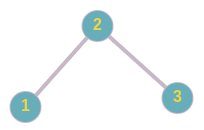5

# Largest BST subtree

Difficulty: EASY
Avg. time to solve
10 min
Success Rate
90%

Problem Statement
Suggest Edit

#### A binary search tree (BST) is a binary tree data structure which has the following properties.

``````• The left subtree of a node contains only nodes with data less than the node’s data.
• The right subtree of a node contains only nodes with data greater than the node’s data.
• Both the left and right subtrees must also be binary search trees.
``````
##### Input Format:
``````The first line contains an Integer 'T' which denotes the number of test cases or queries to be run. Then the test cases follow.

The first line of input contains the elements of the tree in the level order form separated by a single space.

If any node does not have a left or right child, take -1 in its place. Refer to the example below.

Example:

Elements are in the level order form. The input consists of values of nodes separated by a single space in a single line. In case a node is null, we take -1 in its place.

For example, the input for the tree depicted in the below image would be :
````````````1
2 3
4 -1 5 6
-1 7 -1 -1 -1 -1
-1 -1

Explanation :
Level 1 :
The root node of the tree is 1

Level 2 :
Left child of 1 = 2
Right child of 1 = 3

Level 3 :
Left child of 2 = 4
Right child of 2 = null (-1)
Left child of 3 = 5
Right child of 3 = 6

Level 4 :
Left child of 4 = null (-1)
Right child of 4 = 7
Left child of 5 = null (-1)
Right child of 5 = null (-1)
Left child of 6 = null (-1)
Right child of 6 = null (-1)

Level 5 :
Left child of 7 = null (-1)
Right child of 7 = null (-1)

The first not-null node (of the previous level) is treated as the parent of the first two nodes of the current level. The second not-null node (of the previous level) is treated as the parent node for the next two nodes of the current level and so on.

The input ends when all nodes at the last level are null (-1).
``````
##### Note :
``````The above format was just to provide clarity on how the input is formed for a given tree.

The sequence will be put together in a single line separated by a single space. Hence, for the above-depicted tree, the input will be given as:

1 2 3 4 -1 5 6 -1 7 -1 -1 -1 -1 -1 -1
``````
##### Output Format :
``````For each test case, print an integer denoting the largest size of the subtree of the binary tree which is also a BST.
``````
##### Note:
``````You do not need to print anything; it has already been taken care of. Just implement the given function.
``````
##### Constraints:
``````1 <= T <= 100
1 <= N <= 5000
1 <= data <= 10^5 and data!=-1

Time Limit: 1sec
``````
##### Sample Input 1 :
``````2
5 2 4 1 3 -1 -1 -1 -1 -1 -1
2 1 3 -1 -1 -1 -1
``````
##### Sample Output 1:
``````3
3
``````

#### Explanation for Sample 1:

``````The BST corresponding to the first test case is-
````````````The subtree rooted at 2 is a BST and its size is 3.

The BST corresponding to the second test case is -
````````````The subtree rooted at 2 is a BST and its size is 3.
``````
##### Sample Input 2 :
``````1
50 -1 20 -1 30 -1 40 -1 50 -1 -1
``````
##### Sample Output 2:
``````4
``````Console